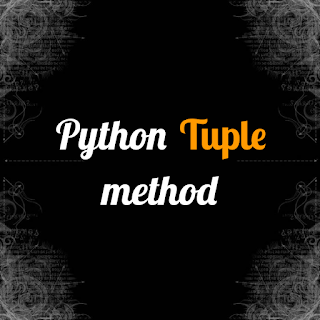# Python Tuple Method - codebugfreePython tuple method

Python tuple is the difference types of Datatypes like Dictionary set List. In this section we will learn the method and function of the Tuple. Tuple is rare use by programmer.

Tuple is mainly not use by a python programmer because. Tuple has does not change the value if  second time. It means tuple is not access able. Tuple is use to the one time run program or in fucture no need to update the some value including in tuple.

This is the main reason the tuple is not use by the Programmer. Programmer wants to update the program in future so the tuple is not access able and does not update the tuple value if ones time value assign than value doesn't change.

We will see the tuple of python code. See the error what happen when we update the value of tuple. see the error and describe the error. Also describe the  index function use in tuple datatypes.

Short description of Index is the built in function of the python. It calculate the given number of the Placement Don't worry we will see all this in code.

Syntax of tuple

``````Make_tuple = (1,1,23,34,32,56,4,1321,236,5,123,76,213,7,3247,6,32,6,6,54,897231,65,23,)
print("It is the given tuple value", Make_tuple)``````

It is the syntax of the Tuple. In tuple we use the Curly bracket () It is the sing of tuple. MOst beginner programmer is confused in sing of the bracket of all datatypes.

INOUTPUT

``It is the given tuple value (1, 1, 23, 34, 32, 56, 4, 1321, 236, 5, 123, 76, 213, 7, 3247, 6, 32, 6, 6, 54, 897231, 65, 23)``

See what happen When we access the some value and change the assing value from the given tuple.

``````Make_tuple = (1,1,23,34,32,56,4,1321,236,5,123,76,213,7,3247,6,32,6,6,54,897231,65,23,)
print("It is the given tuple value", Make_tuple)
Make_tuple = 45
print(Make_tuple)``````

We access the the tuple value and change the tuple value. Let see in output the value is change or not.

INOUTPUT

`````` Make_tuple = 45
TypeError: 'tuple' object does not support item assignment``````

Now we see the the use of index and working of index function in tuple.

``````Make_tuple = (1,1,23,34,32,56,4,1321,236,5,123,76,213,7,3247,6,32,6,6,54,897231,65,23,)
print("It is the given tuple value", Make_tuple)
checking_index = Make_tuple.index(5)
print(checking_index)``````

INOUTPUT

``````It is the given tuple value (1, 1, 23, 34, 32, 56, 4, 1321, 236, 5, 123, 76, 213, 7, 3247, 6, 32, 6, 6, 54, 897231, 65, 23)
9``````

We give the value 5 in index than the index is see the 5 in the tuple value. In the position of 9 their is 5. Count from 0 not a 1.